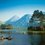Assume to bodies doing circular motion with the same $\omega$.

Case 1 : One body is doing circular motion about point $(0,0,0)$ and path has a radius $R$.The other body is doing circular motion about the point $(0,0,y)$ with radius $R$.

Case 2 : Both bodies doing circular motion about $(0,0,0)$ with different radius $r$ and $R$.

Find the relative angular velocity for both the cases. You sit on the particle with center $(0,0,y)$ for case 1 and on the one with smaller radii ($r) for case 2.

Details and Assumptions

• Take 3 cases of $y > R , y < R , y = R$.
• For Case 1 : The both particles have same $x$ and $y$ coordinate.
• For Case 2 : Both particles and center are co-linear.Note by Rajdeep Dhingra
5 years, 6 months ago

This discussion board is a place to discuss our Daily Challenges and the math and science related to those challenges. Explanations are more than just a solution — they should explain the steps and thinking strategies that you used to obtain the solution. Comments should further the discussion of math and science.

When posting on Brilliant:

• Use the emojis to react to an explanation, whether you're congratulating a job well done , or just really confused .
• Ask specific questions about the challenge or the steps in somebody's explanation. Well-posed questions can add a lot to the discussion, but posting "I don't understand!" doesn't help anyone.
• Try to contribute something new to the discussion, whether it is an extension, generalization or other idea related to the challenge.

MarkdownAppears as
*italics* or _italics_ italics
**bold** or __bold__ bold
- bulleted- list
• bulleted
• list
1. numbered2. list
1. numbered
2. list
Note: you must add a full line of space before and after lists for them to show up correctly
paragraph 1paragraph 2

paragraph 1

paragraph 2

[example link](https://brilliant.org)example link
> This is a quote
This is a quote
    # I indented these lines
# 4 spaces, and now they show
# up as a code block.

print "hello world"
# I indented these lines
# 4 spaces, and now they show
# up as a code block.

print "hello world"
MathAppears as
Remember to wrap math in $$ ... $$ or $ ... $ to ensure proper formatting.
2 \times 3 $2 \times 3$
2^{34} $2^{34}$
a_{i-1} $a_{i-1}$
\frac{2}{3} $\frac{2}{3}$
\sqrt{2} $\sqrt{2}$
\sum_{i=1}^3 $\sum_{i=1}^3$
\sin \theta $\sin \theta$
\boxed{123} $\boxed{123}$

Sort by:

for case 2 - w=ar/R

(w - omega - could not use latex !) (a - the given omega )

- 5 years, 6 months ago

And I think that for case 1 we require their initial x-y positions ......... before they start rotating @Rajdeep Dhingra

- 5 years, 6 months ago

For case 1 ans is 0. For case 2 : I think maybe again 0

- 5 years, 6 months ago

0 - ?

- 5 years, 6 months ago

Yup 0.

- 5 years, 6 months ago